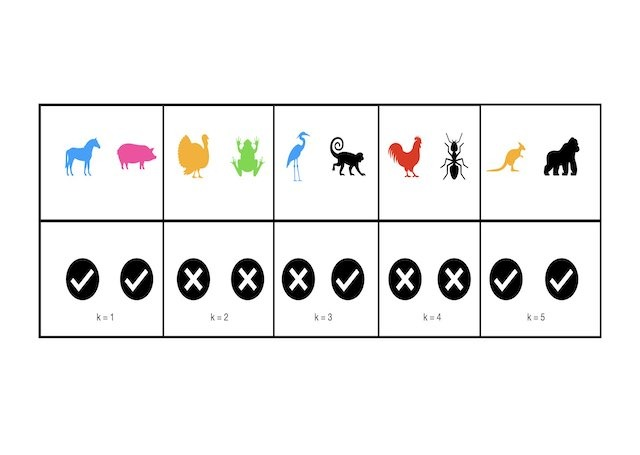# Regression

This category groups articles on the subject of regression. Each post focuses on either a specific regression algorithm, or a tool used when tackling a regression problem. The emphasis here is on understanding these models and techniques at a technical level. Here you will learn to build regression models in Python from scratch.## Can Decision Trees Handle Categorical Features?

Can Decision Trees Handle Categorical Features? Yes, Decision Trees handle categorical features naturally. Often these features are treated by first one-hot-encoding (OHE) in a preprocessing step. However, it is straightforward to extend the CART algorithm to make use of categorical features without such preprocessing. In this post, I will implement classification and regression Decision Trees capable …## Can Decision Trees Handle Missing Values?

Can Decision Trees Handle Missing Values? Yes, Decision Trees handle missing values naturally. It is straightforward to extend the CART algorithm to support the handling of missing values. However, attention needs to be made regarding how the algorithm is implemented in code. In this post, I will implement classification and regression Decision Trees capable of dealing …## 3 Methods to Tune Hyperparameters in Decision Trees

3 Methods to Tune Hyperparameters in Decision Trees We can tune hyperparameters in Decision Trees by comparing models trained with different parameter configurations, on the same data. An optimal model can then be selected from the various different attempts, using any relevant metrics. There are several different techniques for accomplishing this task. Three of the …## Implement the KNN Algorithm in Python from Scratch

Implement the KNN Algorithm in Python from Scratch In this post, we will cover the K Nearest Neighbours algorithm: how it works and how it can be used. We will work through implementing this algorithm in Python from scratch, and verify that our model works as expected. What is the KNN Algorithm? K Nearest Neighbours …## Implement Gradient Boosting Regression in Python from Scratch

Implement Gradient Boosting Regression in Python from Scratch In this post, we will implement the Gradient Boosting Regression algorithm in Python. This is a powerful supervised machine learning model, and popularly used for prediction tasks. To gain a deep insight into how this algorithm works, the model will be built up from scratch, and subsequently verified against the …## Understanding the Gradient Boosting Regressor Algorithm

Understanding the Gradient Boosting Regressor Algorithm In this post, we will cover the Gradient Boosting Regressor algorithm: the motivation, foundational assumptions, and derivation of this modelling approach. Gradient boosters are powerful supervised algorithms, and popularly used for predictive tasks. Motivation: Why Gradient Boosting Regressors? The Gradient Boosting Regressor is another variant of the boosting ensemble technique that …## A Complete Introduction to Cross Validation in Machine Learning

A Complete Introduction to Cross Validation in Machine Learning This post will discuss various Cross Validation techniques. Cross Validation is a testing methodology used to quantify how well a predictive machine learning model performs. Simple illustrative examples will be used, along with coding examples in Python. What is Cross Validation? A natural question to ask, when …## Understanding the Adaboost Regression Algorithm

Understanding the Adaboost Regression Algorithm In this post, we will describe the Adaboost regression algorithm. We will start with the basic assumptions and mathematical foundations of this algorithm, and work straight through to an implementation in Python from scratch. Motivation: What is a Adaboost Regressor? In this post, we will describe the Adaboost regression algorithm. We will …